## 一、介绍CubeMap

Cubemap是一个由六个独立的正方形纹理组成的集合，它将多个纹理组合起来映射到一个单一纹理。

CubeMap通常被用来作为具有反射属性物体的反射源。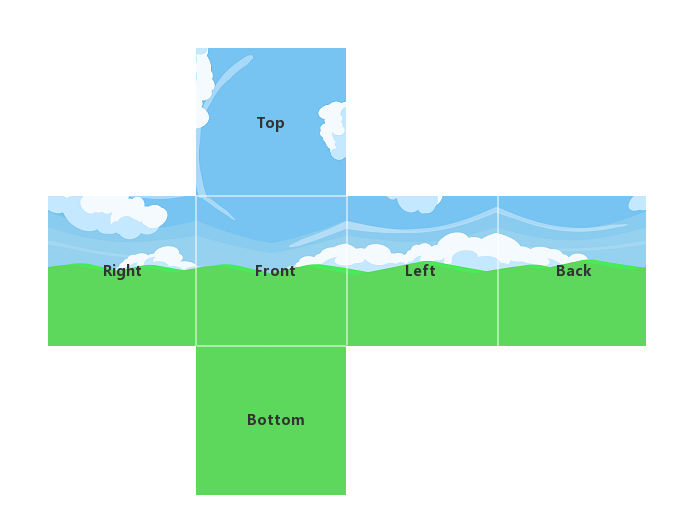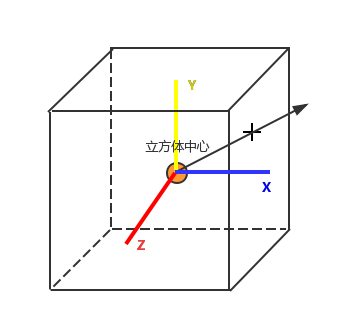``texCUBE(_CubeMap, directionVec);``

texCUBE会采样方向向量directionVec在CubeMap上的交点。

``````Shader "Hidden/CubemapSampler"
{
Properties
{
_CubeMap("CubeMap", CUBE) = ""{}
}
{
Pass
{
CGPROGRAM
#pragma vertex vert
#pragma fragment frag

#include "UnityCG.cginc"

struct appdata
{
float4 vertex : POSITION;
float3 normal : NORMAL;
};

struct v2f
{
float4 vertex : SV_POSITION;
float4 vertexLocal : TEXCOORD1;
};

v2f vert (appdata v)
{
v2f o;
o.vertexLocal = v.vertex;
o.vertex = UnityObjectToClipPos(v.vertex);

return o;
}

samplerCUBE _CubeMap;
fixed4 frag (v2f i) : SV_Target
{
fixed4 col = texCUBE(_CubeMap, normalize(i.vertexLocal.xyz));
return col;
}
ENDCG
}
}
}``````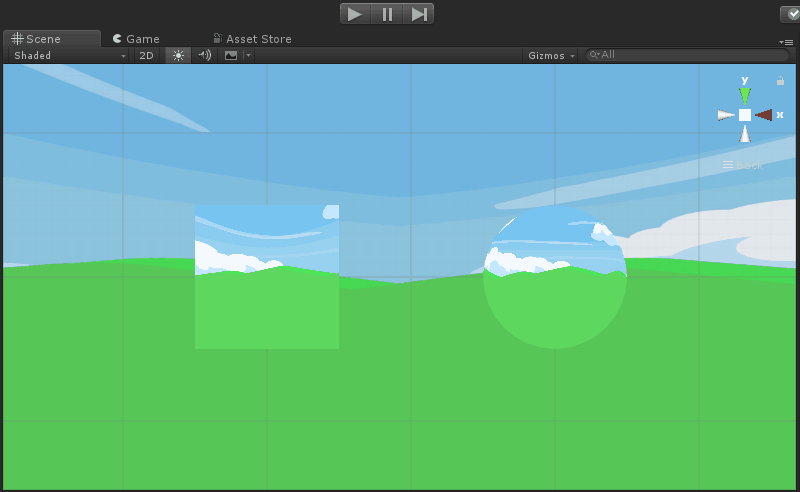### Unity中如何制作CubeMap

1. 已经有6张2D纹理，直接创建CubeMap
Project-Create-Legacy-CubeMap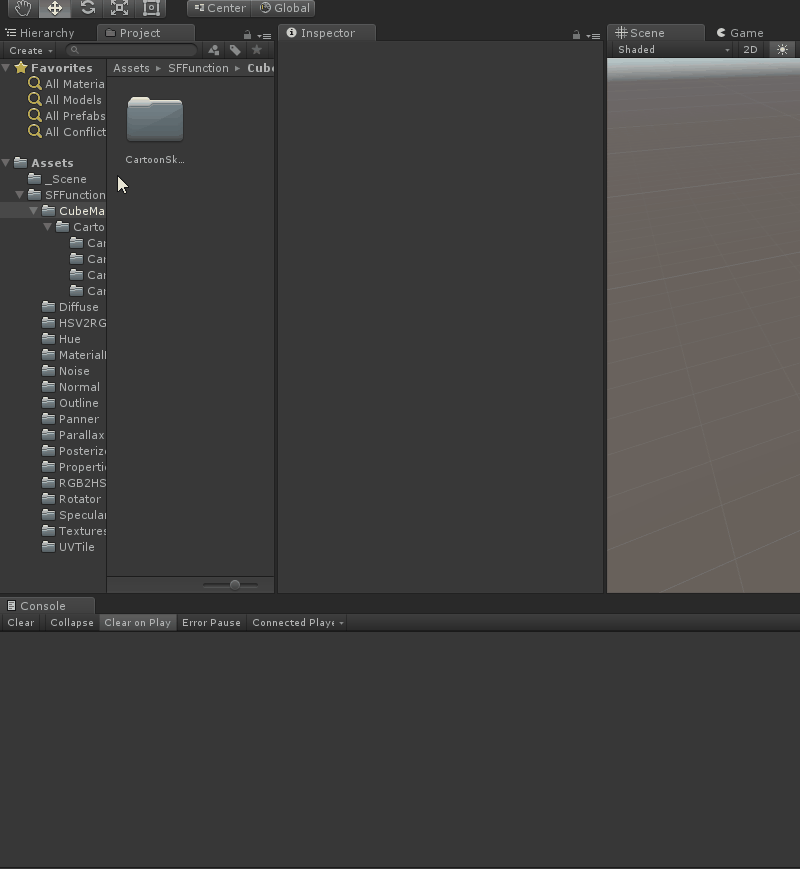## 二、Reflect CubeMap（反射立方体纹理用于环境映射）

### 环境映射原理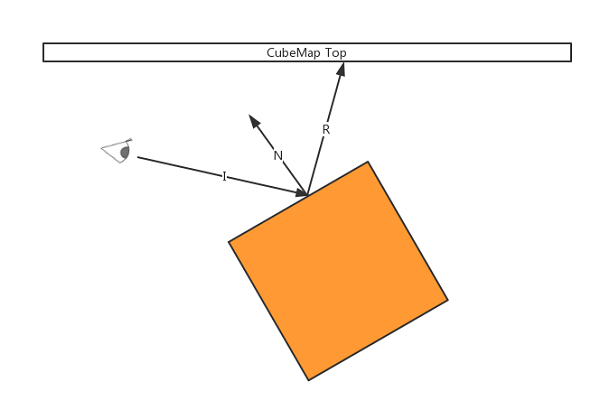### 反射方向的计算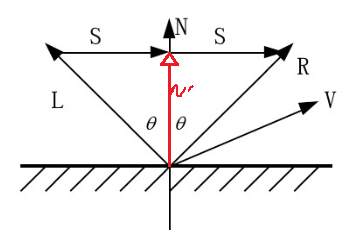L为入射光（顶点到光源）的单位法向量，N为顶点的单位法向量，R为反射光的单位法向量，V是观察方向。

R＝2(N•L)N－L

L在N方向上的投影是|Lcosθ|=cosθ，那么投影矢量N’=Ncosθ
L+S = N’ = Ncosθ
R = L+2S = L+2(Ncosθ-L) = 2Ncosθ-L

float3 R = reflect(I,N);

- 创建用于环境映射的立方体纹理（反射源）
- 用该立方体纹理作为天空盒子
- 使用worldRef（视线关于物体顶点法线的反射向量）作为采样的方向向量，对CubeMap采样作为物体的表面纹理

``````Shader "Hidden/CM       Reflect"
{
Properties
{
_CubeMap("CubeMap", CUBE) = ""{}
}
{
Pass
{
CGPROGRAM
#pragma vertex vert
#pragma fragment frag

#include "UnityCG.cginc"

struct appdata
{
float4 vertex : POSITION;
float3 normal : NORMAL;
};

struct v2f
{
float4 vertex : SV_POSITION;
float3 worldNormal : TEXCOORD0;
float3 worldRef : TEXCOORD1;
float3 worldPos : TEXCOORD2;
float3 worldViewDir : TEXCOORD3;
};

v2f vert (appdata v)
{
v2f o;
o.vertex = UnityObjectToClipPos(v.vertex);
o.worldPos = mul(unity_ObjectToWorld, v.vertex);
o.worldNormal = mul(unity_ObjectToWorld, v.normal);
o.worldViewDir =  normalize(_WorldSpaceCameraPos.xyz - o.worldPos.xyz);
o.worldRef = reflect(-o.worldViewDir,normalize(o.worldNormal));
return o;
}

samplerCUBE _CubeMap;
fixed4 frag (v2f i) : SV_Target
{
fixed4 col = texCUBE(_CubeMap, i.worldRef);
return col;
}
ENDCG
}
}
}``````

#### 反射效果展示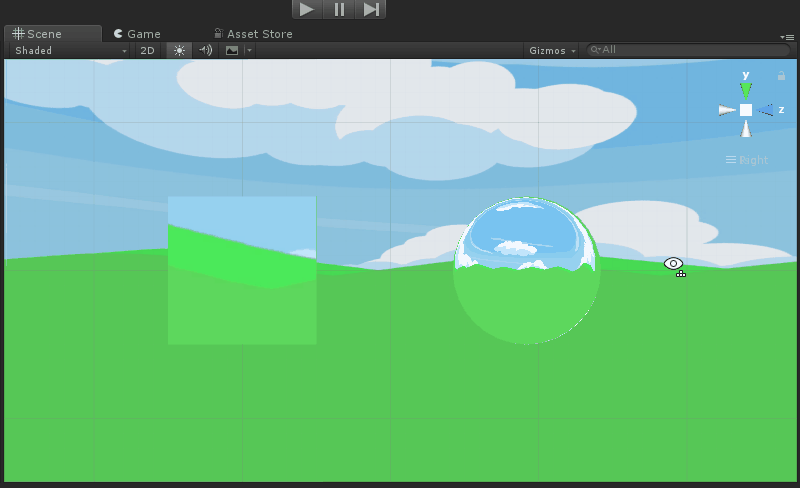### ps:“假”反射？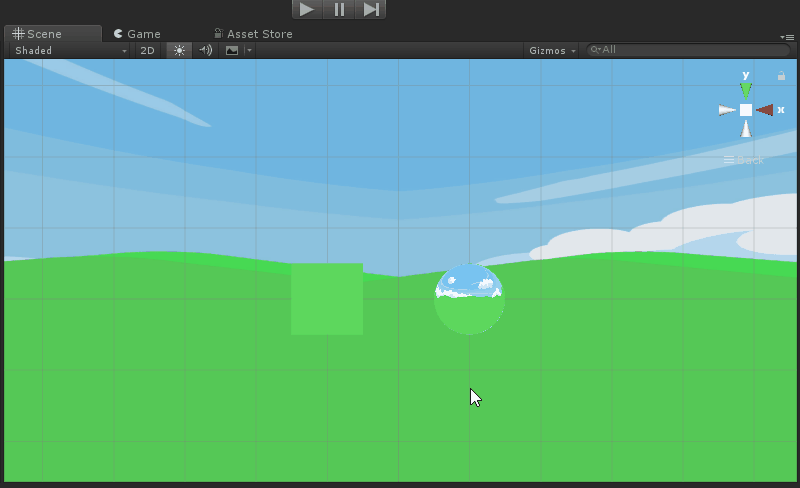#### 一个非常消耗效率的真反射实现方法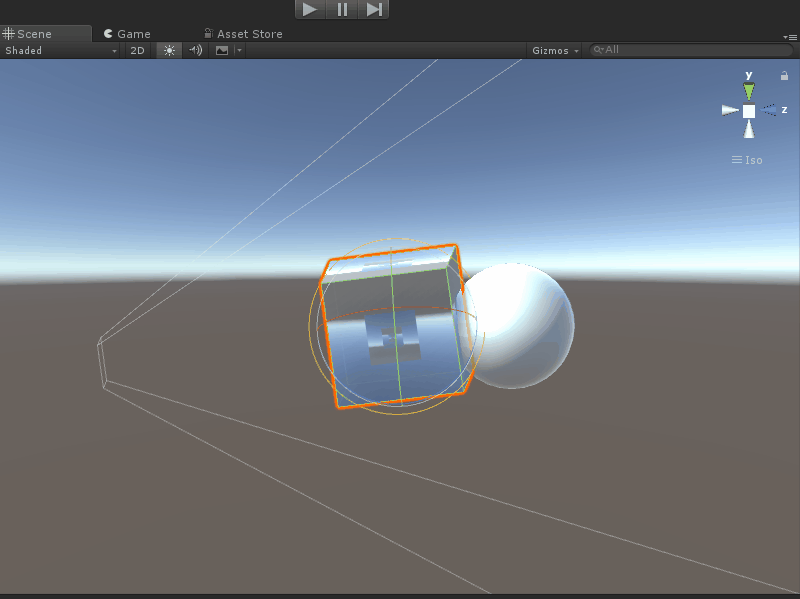## 三、Refract CubeMap（折射立方体纹理用于环境透视）

``texCube(_CubeMap, refract(viewDir,normal,_RefractRatio));``

_RefractRatio是折射相对系数，与物体的介质有关。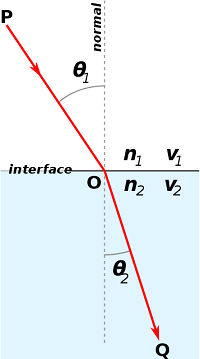sinθ比值等于介质折射率n的比值

1.33
1.309

### 环境透视的实现原理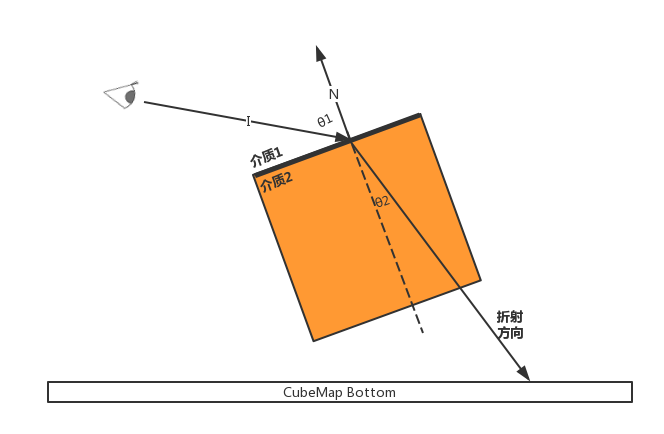``````Shader "Hidden/CMRefract"
{
Properties
{
_CubeMap("CubeMap", CUBE) = ""{}
_RefractRatio("Refract Ratio", Float) = 0.5
}
{
Pass
{
CGPROGRAM
#pragma vertex vert
#pragma fragment frag

#include "UnityCG.cginc"

struct appdata
{
float4 vertex : POSITION;
float3 normal : NORMAL;
};

struct v2f
{
float4 vertex : SV_POSITION;
float3 worldNormal : TEXCOORD0;
float3 worldRef : TEXCOORD1;
float3 worldPos : TEXCOORD2;
float3 worldViewDir : TEXCOORD3;
float4 vertexLocal : TEXCOORD4;
};

float _RefractRatio;
v2f vert (appdata v)
{
v2f o;
o.vertexLocal = v.vertex;
o.vertex = UnityObjectToClipPos(v.vertex);
o.worldPos = mul(unity_ObjectToWorld, v.vertex);
o.worldNormal = mul(unity_ObjectToWorld, v.normal);
o.worldViewDir =  normalize(_WorldSpaceCameraPos.xyz - o.worldPos.xyz);
o.worldRef = refract(-o.worldViewDir,normalize(o.worldNormal),_RefractRatio);
return o;
}

samplerCUBE _CubeMap;
fixed4 frag (v2f i) : SV_Target
{
fixed4 col = texCUBE(_CubeMap, normalize(i.worldRef));
return col;
}
ENDCG
}
}
}``````

#### 折射效果展示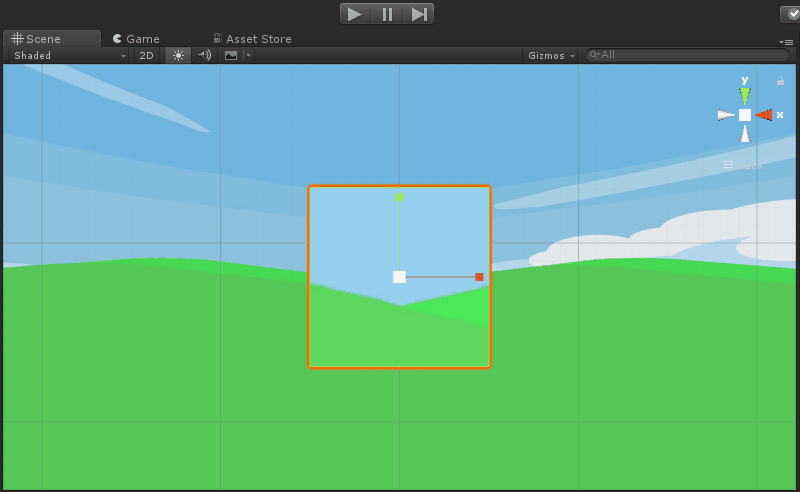## 四、菲涅尔反射（同时考虑折射与反射）

• Schlick菲涅尔近似等式
``````//resnelBias 菲尼尔偏移系数
//fresnelScale 菲尼尔缩放系数
//fresnelPower 菲尼尔指数
reflectFact = fresnelBias + fresnelScale*pow(1-dot(viewDir,N)),fresnelPower);  //系数：多少光发生折射多少光发生反射``````
• Empricial菲涅尔近似等式：
``````//F0是反射系数用于控制菲涅尔的强度
reflectFact = F0 + (1-F0)*pow(1-dot(viewDir,N),5);``````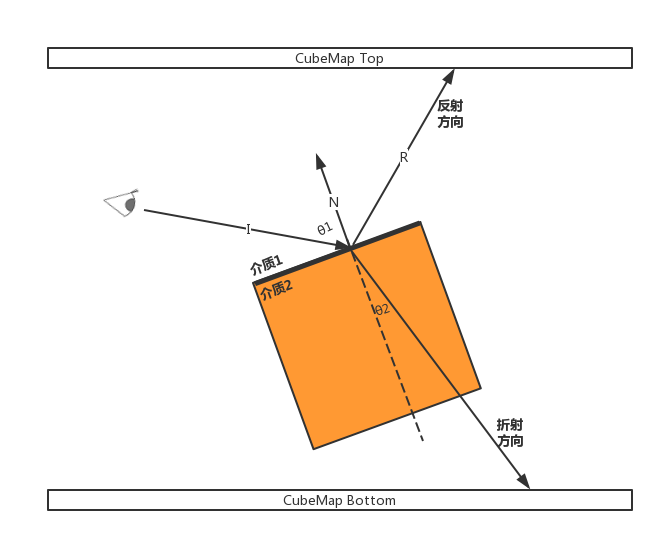``````c(reflect) = reflect(viewDir,N)
C(fresnelFinal) = reflectFact * C(reflect) + (1-reflectFact)*C(refract);  //能量守恒``````

### 以Schlick菲涅尔公式为例实现Fresnel反射

``````Shader "Hidden/Fresnel_Schlick"
{
Properties
{
_CubeMap("CubeMap", CUBE) = ""{}
_RefractRatio("Refract Ratio", Float) = 0.5
_FresnelScale("Fresnel Scale", Float) = 0.5
}
{
Pass
{
CGPROGRAM
#pragma vertex vert
#pragma fragment frag

#include "UnityCG.cginc"

struct appdata
{
float4 vertex : POSITION;
float3 normal : NORMAL;
};

struct v2f
{
float4 vertex : SV_POSITION;
float3 worldNormal : TEXCOORD0;
float3 worldReflect : TEXCOORD1;
float3 worldPos : TEXCOORD2;
float3 worldViewDir : TEXCOORD3;
float4 vertexLocal : TEXCOORD4;
float3 worldRefract : TEXCOORD5;
};

float _RefractRatio;
v2f vert (appdata v)
{
v2f o;
o.vertexLocal = v.vertex;
o.vertex = UnityObjectToClipPos(v.vertex);
o.worldPos = mul(unity_ObjectToWorld, v.vertex);
o.worldNormal = mul(unity_ObjectToWorld, v.normal);
o.worldViewDir =  normalize(_WorldSpaceCameraPos.xyz - o.worldPos.xyz);
o.worldReflect = reflect(-o.worldViewDir,normalize(o.worldNormal));
o.worldRefract = refract(-o.worldViewDir,normalize(o.worldNormal),_RefractRatio);
return o;
}

float _FresnelScale;
samplerCUBE _CubeMap;
fixed4 frag (v2f i) : SV_Target
{
float4 fresnelReflectFactor = _FresnelScale + (1 - _FresnelScale)*pow(1-dot(i.worldViewDir,i.worldNormal), 5);
fixed4 colReflect = texCUBE(_CubeMap, normalize(i.worldReflect));
fixed4 colRefract = texCUBE(_CubeMap, normalize(i.worldRefract));
fixed4 col = fresnelReflectFactor * colReflect + (1-fresnelReflectFactor) * colRefract;
return col;
}
ENDCG
}
}
}``````

#### 菲涅尔反射效果展示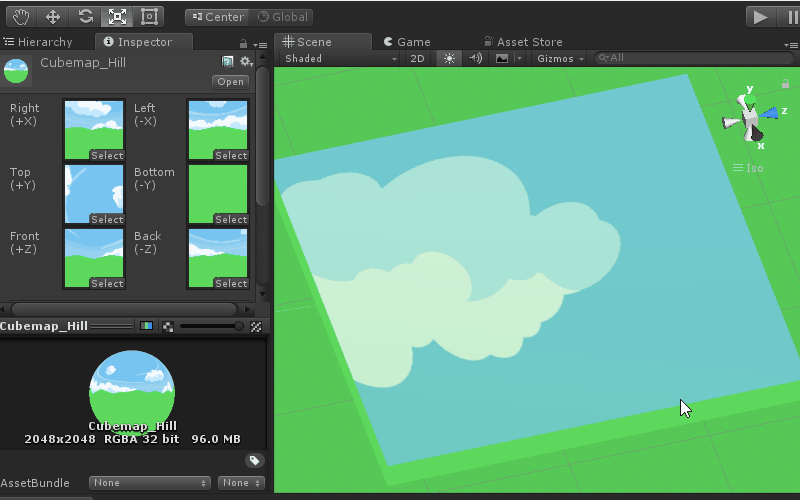## 参考

### iis 搭建ftp服务器_weixin_38095675的博客-程序员宝宝

1、通过控制面板打开 windows 功能2、搜索iis，进入iis服务管理器3、选择网站，右键添加 ftp 站点---填写ftp服务器名、物理路径4、填写ftp服务器ip地址（选择本机地址）、ssl选择无5、填写身份验证，注意此处选择指定用户，填写的用户名需要与cmd命令窗口用户一致，不然登录时候总是填写不对用户名和密码，点击完成6、测试是否成功ftp://192...